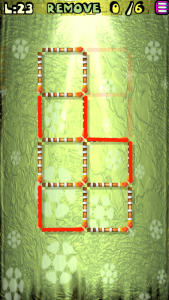# Move the Matches Puzzles Episode 2 Level 21-40 Walkthrough

Walkthrough for all the solutions and answers to the game Move the Matches Puzzles by K-Lab. Get the app on the Google Play Store to play along if you do not already have it.  This is for episode 2, levels 21 through 40.

### Level 21Instructions: Move 2 matches to get 4 squares

### Level 22Instructions: Add 2 matches to get the correct equation

Solution: 1+8=9

### Level 23Instructions: Remove 6 matches to get 3 squares, all equal

### Level 24Instructions: Move 1 match to correct the equation

Solution: 4/2=2

### Level 25Instructions: Remove 2 matches to correct the equation

Solution: 3/1=3

### Level 26Instructions: Remove 4 matches to get 4 squares, all equal

### Level 27Instructions: Move 4 matches to get 4 squares

### Level 28Instructions: Move 2 matches to correct the equation

Solution: 2×5=10

### Level 29Instructions: Remove 2 matches to correct the equation

Solution: 3×5=15

### Level 30Instructions: Add 2 matches to get 5 squares

### Level 31Instructions: Move 2 matches to correct the equation

Solution: 3×4=12

### Level 32Instructions: Move 2 matches to correct the equation

Solution: 4+8=12

### Level 33Instructions: Remove 2 matches to correct the equation

Solution: 5+5=10

### Level 34Instructions: Remove 2 matches to correct the equation

Solution: 7-4=3

### Level 35Instructions: Add 4 matches to get 3 squares

### Level 36Instructions: Remove 1 match to correct the equation

Solution: 5+8=13

### Level 37Instructions: Add 2 matches to get 3 squares

### Level 38Instructions: Remove 4 matches to get 3 triangles

### Level 39Instructions: Add 3 matches to correct the equation

Solution: 3+6=9

### Level 40Instructions: Remove 4 matches to get 3 triangles